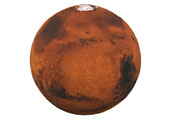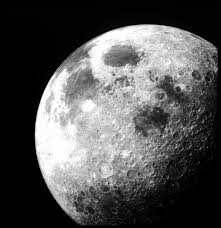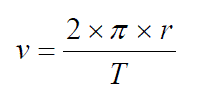Edexcel IGCSE - Astronomy

Page 1

 NEED HELP? Edexcel iGCSE digital textbooks From the makers of GradeGorilla. GGebooks.com (Opens in a new tab)1. Mars has a smaller gravitational field strength at the surface than the Earth. This is because...

• A) the mass of Mars is smaller than Earth.
• B) Mars has a very thin atmosphere.
• C) Mars has no water on the surface.
• D) Mars orbits the Sun at a slower speed than Earth.2. Which of the following answers best describes the orbits of planets, moons and comets?

 Nearly circular orbit Elliptical (non-circular) orbit A. planets moons, comets B. planets, moons comets C. comets, moons planets D. moons comets, planets

3. Which of the following gives the sizes of astronomical objects in order of increasing size?

• A) Solar system, universe, galaxy.
• B) Universe, galaxy, solar system.
• C) Solar system, galaxy, universe.
• D) Galaxy, solar system, universe.

4. Approximately how many stars are in a typical galaxy?

• A) Hundreds of stars.
• B) thousands of stars.
• C) millions of stars.
• D) billions of stars.

5. What is the name of our own galaxy containing the Sun?

• A) The Andromeda galaxy.
• B) The Milky Way galaxy.
• C) The Orion galaxy.
• D) The Hubble galaxy.

6. Which of these statements is correct?

• A). Planets orbit moons, planets orbit stars.
• B). Moons orbit planets, stars orbit planets.
• C). Moons orbit stars, stars orbit planets.
• D). Moons orbit planets, planets orbit stars.7. The formula on the right can be used to calculate the orbital velocity v of any object. It can be re-arranged to find the radius of the orbit.Which of the following has been correctly re-aranged?8. The radius of the Earth's orbit is about 150 million kilometres. In standard form, and given in metres, this is ..

• A). 1.5 x 108 m
• B). 1.5 x 1010 m
• C). 1.5 x 1011 m
• D). 1.5 x 1012 m

9. The Earth orbits the Sun in 1 year, which is 3.2 x 107 seconds. Using this figure, along with information given in questions 7 and 8, what is the orbital velocity of the Earth?

• A). 4.6 x 103 m/s
• B). 29 m/s 103
• C). 4.6 m/s 103
• D). 2.9 x 104 m/s